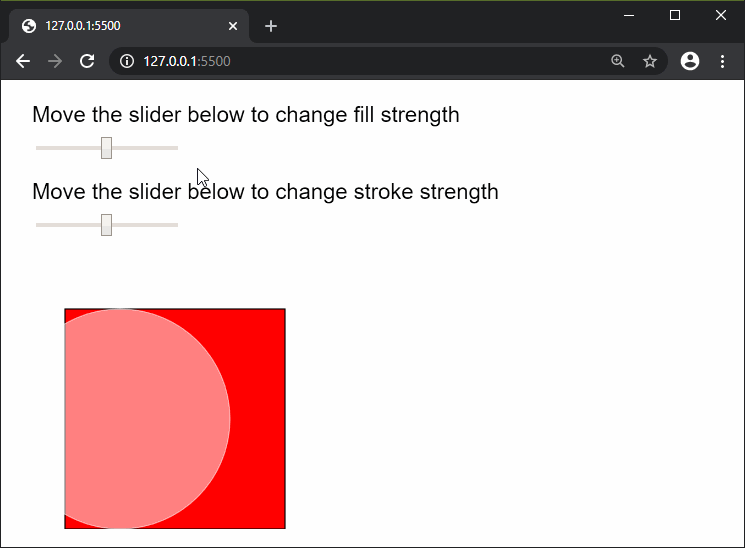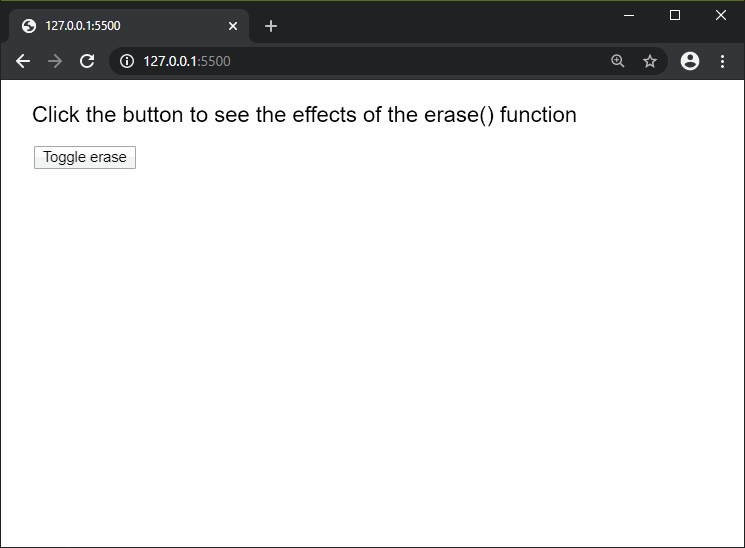Related Articles
p5.js | erase() Function
• Last Updated : 24 Apr, 2020

The erase() function in p5.js is used to subtract all the drawings that would be done after the use of this function. The subtracted drawing area would reveal the webpage below the canvas. The effect of this function can be canceled by using the noErase() function.

It does not affect the drawings done by the image() and background() functions.

Syntax:

`erase( [strengthFill], [strengthStroke] )`

Parameters: This function accept two parameters as mentioned above and described below.

• strengthFill: It is a number in the range of 0 to 255, which denotes the strength of the erasing shape’s fill. It is an optional value. The default value is 255, which denotes the full strength of the shape’s fill.
• strengthStroke: It is a number in the range of 0 to 255, which denotes the strength of the erasing shape’s stroke. It is an optional value. The default value is 255, which denotes the full strength of the shape’s stroke.

Below examples illustrate the erase() function in p5.js:

Example 1:

 `function` `setup() {``  ``createCanvas(600, 400);``  ``textSize(20);`` ` `  ``fill(``'black'``);``  ``text(``"Move the slider below to change fill strength"``, 20, 30);`` ` `  ``fillStrengthSlider = createSlider(0, 255, 128, 1);``  ``fillStrengthSlider.position(30, 50);`` ` `  ``text(``"Move the slider below to change stroke strength"``, 20, 100);`` ` `  ``strokeStrengthSlider = createSlider(0, 255, 128, 1);``  ``strokeStrengthSlider.position(30, 120);``}`` ` `function` `draw() {``  ``fill(``'red'``);``  ``rect(50, 200, 200, 200);`` ` `  ``erase(fillStrengthSlider.value(), strokeStrengthSlider.value());``  ``circle(100, 300, 200);``  ``noErase();`` ` `}`

Output:Example 2:

 `let eraseEnable = ``false``;`` ` `function` `setup() {``  ``createCanvas(600, 400);``  ``textSize(20);`` ` `  ``fill(``'black'``);``  ``text(``"Click the button to see the effects of"``         ``+ ``" the erase() function"``, 20, 30);`` ` `  ``toggleBtn = createButton(``"Toggle erase"``);``  ``toggleBtn.position(30, 60);``  ``toggleBtn.mouseClicked(toggleErase);``}`` ` `function` `toggleErase() {``  ``if` `(eraseEnable) {``    ``noErase();``    ``eraseEnable = ``false``;``  ``}``  ``else` `{``    ``erase();``    ``eraseEnable = ``true``;``  ``}``}`` ` `function` `mouseMoved() {``  ``fill(``'red'``);``  ``noStroke();``  ``circle(mouseX, mouseY, 50);``}`

Output:Reference: https://p5js.org/reference/#/p5/eraseMy Personal Notes arrow_drop_up